• +91 9971497814
• info@interviewmaterial.com

# RD Chapter 7- Introduction to Euclid-s Geometry Ex-7.2 Interview Questions Answers

### Related Subjects

Question 1 :
Write two solutions for each of the following equations
(i) 3x + 4y = 7
(ii) x = 6y
(iii) x + πy = 4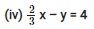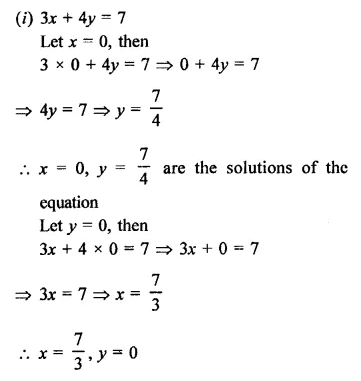(ii)  x = 6y
Let y = 0, then
x = 6 x 0 = 0
∴ x = 0, y = 0
x = 0, y = 0 are the solutions of the equation
Let y= 1, then
x = 6 x 1 = 0                          –
∴ x = 6, y = 1 are the solutions of the equation.
(iii) x + πy = 4
Let x = 4, then
4 + πy = 4
⇒ πy = 4- 4 = 0
∴ y = 0
∴ x = 4, y = 0 are the solutions of the equation
Let x = 0, then
0 + πy = 4 ⇒ πy = 4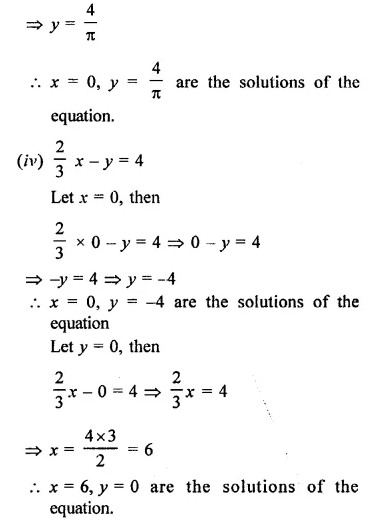Question 2 :
Check which of the following are solutions of the equations 2x – y =6 and which are not
(i) (3, 0)
(ii) (0, 6)
(iii) (2,-2)
(iv)(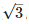,0)
(v) (1/2 ,-5)

Equationis 2x – y = 6
(i) Solution is (3, 0) i.e. x =3, y = 0
Substituting the value of x and y in the equation
2 x 3 – 0 = 6
6 – 0= 6
6 = 6
Which is true
(3, 0) is the solutions.
(ii) (0, 6) i.e. x =0, y =6
Substituting the value of x and y in the equation
2 x 0 – 6 = 6
0-6 = 6
-6 =6  which is not true
(0, 6) is not its solution’
(iii) (2, -2) i.e. x = 2, y =-2
Substituting the value of x and y in the equation
2 x 2 – (-2) = 6
4 + 2= 6
6 = 6which is true.
(2, -2) is the solution.
(iv) (,0) i.e. x =, y = 0,

Substituting the value of x and y in the equations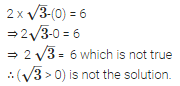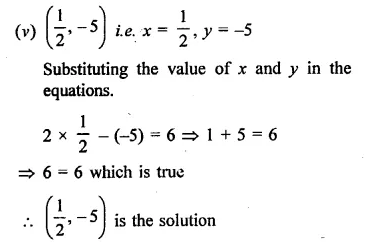Question 3 : If x = -1, y = 2 is a solution of the equation 3x + 4y =k  Find the value of k.

x = -1, y = 2
The equation is 3x + 4y = k
Substituting the value of x and y in it
3 x (-1) + 4 (2) = k
⇒ -3+ 8 = k
⇒  5 = k
∴ k = 5

Question 4 : Find the value of λ if x = -λ and y =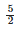is a solution of the equation x + 4y – 7 = 0.

x= -λ, y=Equation is x + 4y – 7 = 0
Substituting the value of x and y,
-λ  + 4 x-7 = 0

-λ + 10 – 7 = 0
-λ +3 = 0
-λ = -3
λ = 3
Hence λ = 3

Question 5 : If x = 2α + 1 and y = α – 1 is a solution of the equation 2x – 3y + 5 = 0, find the value of α.

x = 2α + 1, y = α – 1
are the solution of the equation 2x – 3y + 5 – 0
Substituting the value of x and y
2(2α + 1) -3 (α – 1) + 5 = 0
⇒  4α+ 2-3α+ 3 + 5 = 0
⇒ α+10 = 0
⇒ α = -10
Hence α = -10

Question 6 : If x = 1, and y = 6 is a solution of the equation 8x – ay + a2 = 0, find the values of a.

x = 1, y = 6 is a solution of the equation
8x – ay + a2 = 0
Substituting the value of x and y,
8 x 1-a x 6 + a2 = o
8 – 6a + a2 = 0
a2 – 6a + 8 = 0
a2 – 2a -4a + 8 = 0
a (a – 2) – 4 (a – 2) = 0
(a – 2) (a – 4) = 0
Either a – 2 = 0, then a = 2
or a – 4 = 0, then a = 4
Hence a = 2, 4

Question 7 :
Write two solutions of the form x = 0, y = a and x = b, y = 0 for each of the following equations.
(i) 5x – 2y = 10
(ii) -4x + 3y = 12
(iii) 2x + 3y = 24

(i)  5x – 2y = 10
Let x = 0, then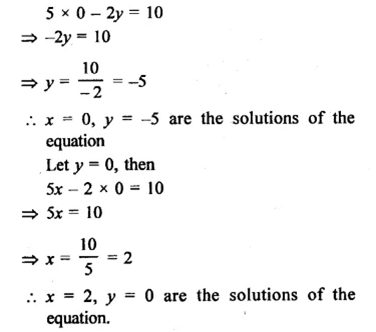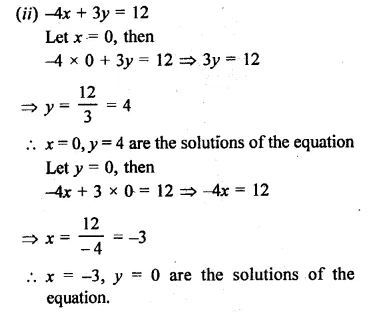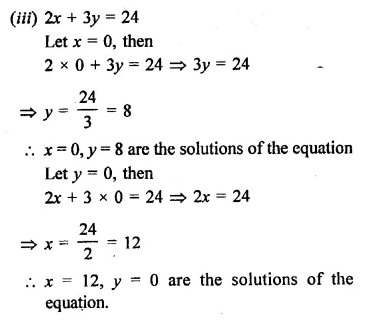Todays Deals### RD Chapter 7- Introduction to Euclid-s Geometry Ex-7.2 Contributorskrishan

Name:
Email:

# Latest News# 9000 interview questions in different categories